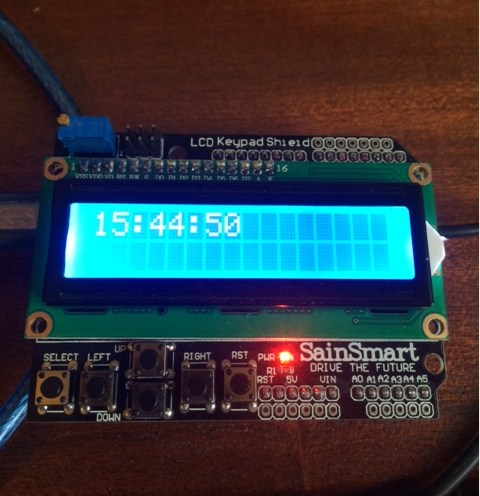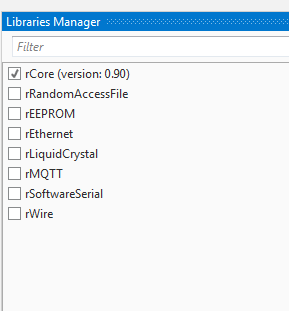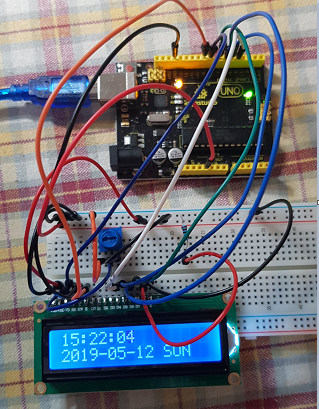# B4R TutorialLCD Clock example

Arduino Uno with LCD shield:Working with a LCD monitor:
1. Initialize the LiquidCrystal object and set the connected pins.
B4X:
``lcd.Initialize(8, 255, 9, Array As Byte (4, 5, 6, 7))``
2. Call Begin with the number of columns and rows:
B4X:
``lcd.Begin(16, 2)``
3. Call Write to print to the LCD. The message can be made of a string, a number or an array of bytes.
You can call Write multiple times.

This example is a bit more complicated.
It works together with the "external serial connector": https://www.b4x.com/android/forum/threads/tool-external-serial-connector.65724/ (a B4J desktop program).

Once the PC is connected to the Arduino, the PC sends the current time (there is no clock on the Arduino).

B4R code:
B4X:
``````Sub AStream_NewData (Buffer() As Byte)
If Buffer.Length <> 3 Then
Log("invalid data: ", Buffer)
Else
hours = Buffer(0)
minutes = Buffer(1)
seconds = Buffer(2)
lcd.Clear
timer1.Enabled = True
End If
End Sub``````

The Arduino starts a timer that shows the current time:
B4X:
``````Sub Timer1_Tick
seconds = seconds + 1
If seconds = 60 Then
seconds = 0
minutes = minutes + 1
End If
If minutes = 60 Then
minutes = 0
hours = hours + 1
End If
If hours = 24 Then hours = 0
lcd.SetCursor(0, 0)
lcd.Write(NumberFormat(hours, 2, 0))
lcd.Write(":")
lcd.Write(NumberFormat(minutes, 2, 0))
lcd.Write(":")
lcd.Write(NumberFormat(seconds, 2, 0))
End Sub``````

The PC can be disconnected afterwards.

The serial connector program used is slightly modified. It sends the time with this code:
B4X:
``````Sub Send_Time
Dim now As Long = DateTime.Now
astream.Write(Array As Byte(DateTime.GetHour(now), DateTime.GetMinute(now), DateTime.GetSecond(now)))
End Sub``````
It calls this sub 5 seconds after the connection with the help of CallSubPlus.

The two projects are attached.

#### Attachments

• B4J_SerialConnector.zip
4.1 KB · Views: 655
• B4R_LCD.zip
987 bytes · Views: 821

#### Cableguy

##### Expert
Longtime User
Hum... I have a .96" 128x96 pixel display (4 wire i2c), can I use this lib with it?

Staff member
Longtime User

#### Moch Firmansyah

##### Member
Longtime User
Can we use existing arduino I2C library for our B4R project, Erel?

#### Erel

##### B4X founder
Staff member
Longtime User
Please start a new thread for any question in the questions forum.

#### miker2069

##### Active Member
Longtime User
Is this a built in library in B4R or a custom library that needs to be installed?

#### Erel

##### B4X founder
Staff member
Longtime User
You can see it in the libraries tab:#### cloner7801

##### Active Member
Longtime User
how to write in row 2 ?

#### Erel

##### B4X founder
Staff member
Longtime User
B4X:
``````lcd.SetCursor(0, 1)
lcs.Write("Second line")``````

•cloner7801

#### Johan Schoeman

##### Expert
Longtime User
I understand that this is an old thread but for a dummy like me that just started with B4R it is very new and just so much fun!

Using @Erel's B4J and B4R projects in post #1, I have managed to get it working on a 1602 LCD display (no shield) and added code to also display the current date and the day of the week (i.e SUN, MON, etc) on line 2 of the LCD display.

This is the only Sub that I have changed in @Erel's B4J code project (the only change needed was in sub Send_Time of the Main module):
B4X:
``````Sub Send_Time
Dim now As Long = DateTime.Now
Dim year As Int = DateTime.GetYear(now)
Dim century As Byte = year/100
Dim yearofcenturay As Byte = year Mod (century * 100)
astream.Write(Array As Byte(DateTime.GetHour(now), DateTime.GetMinute(now), DateTime.GetSecond(now),century, yearofcenturay, DateTime.GetMonth(now), DateTime.GetDayOfMonth(now), DateTime.GetDayOfWeek(now)))
End Sub``````

This is the changes I made to the B4R code:
B4X:
``````#Region Project Attributes
.....
.....
.....
.....

Private hours, minutes, seconds, year1, year2, month, dayofmonth, dayofweek As Byte
.....
.....
.....

End Sub

.....
.....

Sub Timer1_Tick
seconds = seconds + 1
If seconds = 60 Then
seconds = 0
minutes = minutes + 1
End If
If minutes = 60 Then
minutes = 0
hours = hours + 1
End If
If hours = 24 Then hours = 0
lcd.SetCursor(0, 0)
lcd.Write(NumberFormat(hours, 2, 0))
lcd.Write(":")
lcd.Write(NumberFormat(minutes, 2, 0))
lcd.Write(":")
lcd.Write(NumberFormat(seconds, 2, 0))

lcd.SetCursor(0, 1)
lcd.Write(NumberFormat(year1, 2, 0))
lcd.Write(NumberFormat(year2, 2, 0))
lcd.Write("-")
lcd.Write(NumberFormat(month, 2, 0))
lcd.Write("-")
lcd.Write(NumberFormat(dayofmonth, 2, 0))
lcd.Write(" ")
Select dayofweek
Case 1
lcd.Write("SUN")
Case 2
lcd.Write("MON")
Case 3
lcd.Write("TUE")
Case 4
lcd.Write("WED")
Case 5
lcd.Write("THU")
Case 6
lcd.Write("FRI")
Case 7
lcd.Write("SAT")
End Select

End Sub

Sub AStream_NewData (Buffer() As Byte)
If Buffer.Length <> 8 Then
Log("invalid data: ", Buffer)
Else
hours = Buffer(0)
minutes = Buffer(1)
seconds = Buffer(2)
year1 = Buffer(3)
year2 = Buffer(4)
month = Buffer(5)
dayofmonth = Buffer(6)
dayofweek = Buffer(7)
lcd.Clear
timer1.Enabled = True
End If
End Sub

.....
.....
.....``````

For the B4J project in post #1 you need to have library jReflection installed and enabled in the B4J IDE.

Run the B4R project first. Then run the B4J project and select and connect to the port (in the B4J displayed Form/UI) that your Arduino is connect to (I am using an Uno R3).

Schematic of @Erel's project above is something like this:And this is the result that I get:#### Marc DANIEL

##### Active Member#### Attachments

• Horloge.zip
1.5 KB · Views: 110
•klaus, Erel and Johan Schoeman

Replies
0
Views
2K
Replies
0
Views
5K
Replies
1
Views
490
Replies
2
Views
2K
Replies
19
Views
4K